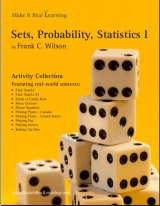^
You are here: HomeMake It Real Learning → Sets, Probability, Statistics I

# Make It Real Learning Sets, Probability, Statistics I workbook46 pages
(10 activities)

Free Sample (PDF):
Rolling the Dice: Using Probability

# Introduction

The Make It Real Learning Sets, Probability, Statistics I workbook focuses on real-world situations that may be effectively analyzed using principles of probability and statistics. This book suits both middle and high school math classrooms.

From determining a winning strategy for a dice game to predicting the contents of a bag of fruit snacks, learners get to use mathematics in meaningful ways. Each activity integrates real world information, companies, and issues (e.g. fruit snacks, Almond Joy, rolling the dice, El Pollo Loco, phone numbers), not just "realistic" data.

There are multiple ways to use the activities of the book in a teaching environment. The activities are an excellent tool for stimulating mathematical discussions in a small group setting. Due to the challenging nature of each activity, group members are motivated to brainstorm problem solving strategies together. The interesting real world contexts motivate them to want to solve the problems. The activities may also be used for individual projects and class-wide discussions.

As a ready-resource for teachers, the workbook also includes completely worked-out solutions for each activity. To make it easier for teachers to assess student work, the solutions are included on a duplicate copy of each activity.

The mathematical objectives of the activities fall within the standards of the National Council of Teachers of Mathematics.

The PDF for this book is enabled for annotation. This means that if you prefer, your student can fill it in on the computer, using the typewriter and drawing tools in Acrobat Reader version 9 or greater.

A review of Math Mammoth and Make It Real Learning Sets, Probability, Statistic by A Day in the Life blog

## Sets, Probability, Statistics I Activity List

Activity TitleMathematical Objectives
Fruit Snacks:
Using Mean, Median, and Mode
Calculate the mean, median, and mode of a data set
Interpret the practical meaning of mean, median, and mode
Fruit Snacks: Using Probability Determine the probability of an event
Kinds of Candy Bars:
Working with Sets
Create a Venn diagram to represent a data set
Find the intersection and union of a group of sets
Find the complement of a set
Working with Combinations
Use combinations to count different outcomes
Phone Numbers:
Using Principles of Counting
Use combinations and permutations to count possible outcomes
Calculate percentages
Using Principles of Counting
Use permutations to count possible outcomes
Use principles of counting
Picking Plates - United States:
Using Permutations
Use permutations to count possible outcomes
Use principles of counting
Playing Pig: Using Expected Value Calculate expected value
Use numerical results to determine a game strategy
Playing Soccer:
Using Combinations and Probability
Use combinations to count different outcomes
Calculate theoretical probabilities
Rolling the Dice: Using Probability Calculate the probability of an event
Calculate conditional probabilities
Use probabilities to develop game strategy

Bundle specials!

Activity Library, Volume I. Price: \$39.99
Includes 11 Make It Real Learning activity workbooks for the price of 8! You get three workbooks for free!

Activity Library, Volume II. Price: \$39.99
Includes 11 Make It Real Learning activity workbooks for the price of 8! You get three workbooks for free!

States by the Numbers Bundle. Price: \$19.99
Inludes all 50 States by the Numbers workbooks at a whopping 87% discount!
(Normal price would be 50 × \$2.99 = \$149.50)

By purchasing any of the Make It Real Learning workbooks, permission IS granted for the teacher (or parent) to reproduce this material to be used with his/her students in a teaching situation; not for commercial resale. However, you are not permitted to share the material with another teacher.

In other words, you are permitted to make copies for the students/children you are teaching, but not for other teachers' usage.

WAIT!

Receive my monthly collection of math tips & resources directly in your inbox — and get a FREE Math Mammoth book!You can unsubscribe at any time.

### Math Mammoth Tour

Confused about the different options? Take a virtual email tour around Math Mammoth! You'll receive:

An initial email to download your GIFT of over 400 free worksheets and sample pages from my books. Six other "TOURSTOP" emails that explain the important things and commonly asked questions concerning Math Mammoth curriculum. (Find out the differences between all these different-colored series!)

This way, you'll have time to digest the information over one or two weeks, plus an opportunity to ask me personally about the curriculum.
A monthly collection of math teaching tips & Math Mammoth updates (unsubscribe any time)### "Mini" Math Teaching Course

This is a little "virtual" 2-week course, where you will receive emails on important topics on teaching math, including:

- How to help a student who is behind
- Troubles with word problems
- Teaching multiplication tables
- Why fractions are so difficult
- The value of mistakes
- Should you use timed tests
- And more!

A GIFT of over 400 free worksheets and sample pages from my books right in the very beginning.A monthly collection of math teaching tips & Math Mammoth updates (unsubscribe any time)
Enter your email to receive math teaching tips, resources, Math Mammoth news & sales, humor, and more! I tend to send out these tips about once monthly, near the beginning of the month, but occasionally you may hear from me twice per month (and sometimes less often).• A GIFT of over 400 free worksheets and sample pages from my books.